by Kirby Urner
First posted: November 9, 1997
Last updated: February 23, 2000

Any tetrahedron may be represented in terms of rays to its four vertices originating from some common point. For example, in the case of the regular tetrahedron, we can simply use the quadray basis set: (1,0,0,0) (0,1,0,0) (0,0,1,0) (0,0,0,1). Unless the origin itself is a vertex, the tetrahedron's set of six edges will not include any of the four vertex-defining vectors. Counting (0,0,0,0) as a zero-vector, we can always net the tetrahedron's edges as six vector subtractions representing the differences between pairs of vertices.

For example, as we learned in An Introduction to Quadrays, the edge between (1,0,0,0) and (0,1,0,0) has a length and direction given by: (1,0,0,0) - (0,1,0,0) = (2,0,1,1). We could also write this difference as (0,1,0,0) - (1,0,0,0) which gives (0,2,1,1), a vector pointing in the opposite direction. However, since this is paper about volume, it is a vector's length, and not its positive versus negative directionality, which proves relevant in our calculations.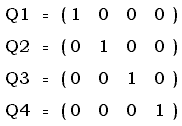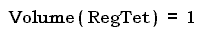For convenience, we can store the four vectors in a matrix, where each row represents a vertex. We can then feed a special case matrix to our volume function, a method defined within our generic tetrahedron class.

Our class method for operating on such a matrix comes from Gerald de Jong. Although aware that Leonhard Euler had come up with an expression for the tetrahedron's volume, given only its six edge lengths as input, Gerald resolved to derive such an expression independently.

Gerald's elegant result, which relates the algebraic terms to open and closed triangles, and opposite edge pairs, made its first public appearance in the form of a Java applet, subsequently modified to accommodate the synergetics approach, which takes our regular "home base" quadray tetrahedron as its unit of volume.

 Given our volume method works for any tetrahedron, we can apply it to determine the volumes of shapes which we assemble from tetrahedra. The icosahedron, for example, consists of 20 tetrahedra defined by the 3 external vertices of each facet, and the origin (0,0,0,0). We have already stored the quadray coordinates of these points in the allpoints table, so it takes only a few lines of code to get an icosahedron volume of ~18.5122959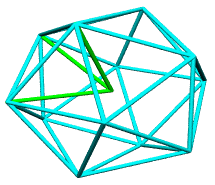The pentagonal dodecahedron is only slightly more complicated in that its 12 regions analyze into 3 tetrahedra apiece. By choosing a corner of the pentagon and drawing two diagonals to opposite vertices, we derive 3 isosceles triangles. The central one is flanked by two which are mirror images of one another, and therefore have the same area. The total volume of the dodecahedron therefore comes to 24 x Outer + 12 x Inner = ~15.3500187
 We might also want to load our matrix with the vertices of an A-module, to confirm that our volume method returns the expected result of 1/24. The A-mod has an edge running from "home base" center to a mid-edge, and another to a mid-face, meaning we need the quadray coodinates for these points. Since the A-mod is part of the concentric hierarchy definition in synergetics, we will add these new coordinates to allpoints and the new edge connections to our shapes table, and confirm a volume of 1/24. ```SHAPEID ID1 ID2 ------------------- A1 ORG AF A1 ORG T1A A1 ORG AE A1 AE AF A1 T1A AF A1 T1A AE AE = midedge AF = midface ORG = (0,0,0,0) T1A = (1,0,0,0) AE = (T1A + T1B)/2 AF = (T1A + T1B + T1C)/3 ```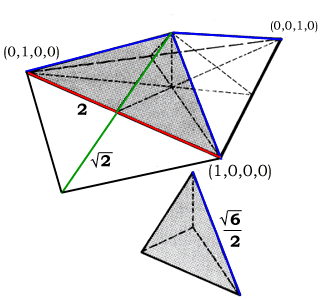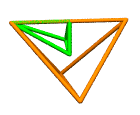David Chako proposed one of the more intriguing implications of using a unit volume tetrahedron within the context of the isotropic vector matrix. He suggested on Synergetics-L that any tetrahedron with vertices at IVM sphere centers would have a whole number volume. An empirical investigation failed to come up with any counter-examples. Bob Gray was able to provide us with a formal proof of Chako's conjecture on August 3, 1997. One way to conceptualize this result is to define the 12 cuboctahedral directions as the Cset -- C for cuboctahedron. Other sets would include the Vset and Eset. The Vset comprises the original 4 quadrays, whereas the Eset consists of all sums of original quadray pairs, which point through the mid-edges of the home tetrahedron -- E stands for edges. Picture four turtles starting from (0,0,0,0). A clock starts ticking off time intervals, and at each tick, the turtles each get to choose one of the 12 Cset directions. Turtle1, for example, might hop (2,0,1,1) in the first interval, and (2,1,0,1) in the second, bringing it to (4,1,1,2). This could go on for a long time, say n ticks. We can symbolize where Turtle1 ends up using the expression below, where the Greek letter sigma simply means the sum of all n hops, where each hop is a random selection from the Cset.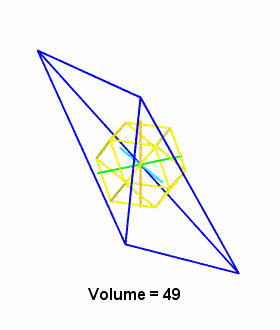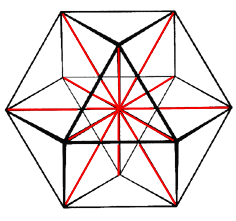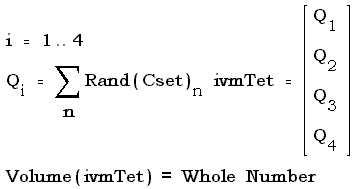One consequence of the above is that any shape assembled from various ivmTets will likewise have a whole number volume. This gives us a lot of freedom to define complicated components which nevertheless have simply expressed volumetric relationships. The vertices of five-fold symmetric shapes do not align with IVM sphere centers however, and cannot be modularized using whole numbers of A and B modules. Instead we make use of the T-module, 1/120th of the rhombic triacontahedron, to map this territory. David Koski's explorations have done the most to disclose the T-mod's ability to serve as the primary module in the world of five-fold symmetric shapes. Koski takes a T-mod and scales it up and down by a constant increment, providing a range of sizes, and shows how he can precisely fill a pentagonal dodecahedron, icosahedron, and many other familiar shapes using whole number combinations of these differently sized T-modular "measuring cups". He even shows how the T-mod itself recursively self-divides into an endless series of smaller and smaller T-mods -- or a finite series plus a "remainder tet" terminator. For further reading: Synergetics on the Web maintained by Kirby Urner